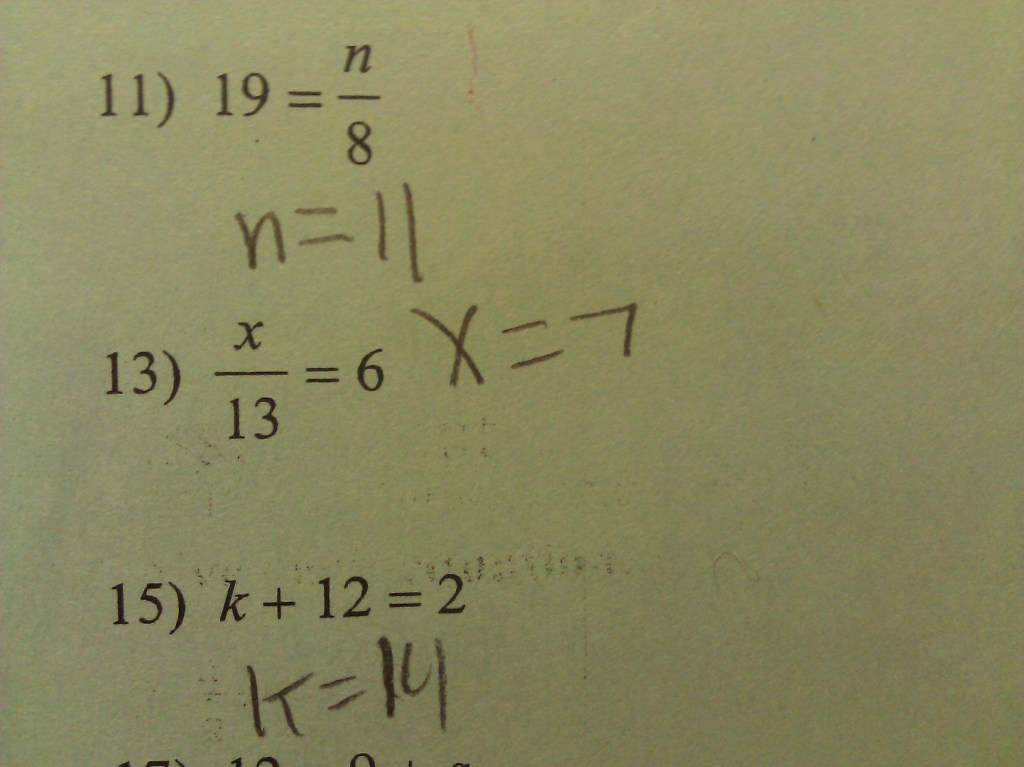# Equations and Inequalities

## Objective

Solve one-step equations with rational numbers.

## Common Core Standards

### Core Standards

?

• 7.EE.B.4.A — Solve word problems leading to equations of the form px + q = r and p(x + q) = r, where p, q, and r are specific rational numbers. Solve equations of these forms fluently. Compare an algebraic solution to an arithmetic solution, identifying the sequence of the operations used in each approach. For example, the perimeter of a rectangle is 54 cm. Its length is 6 cm. What is its width?

?

• 6.EE.B.5

• 6.EE.B.7

## Criteria for Success

?

1. Define and understand a solution to an equation as a number for a variable that makes the equation a true statement when substituted in.
2. Solve one-step equations with rational numbers using addition, subtraction, multiplication, or division.
3. Write and solve one-step equations for contextual situations.

## Tips for Teachers

?

This lesson bridges a gap from 6.EE.7 to 7.EE.4a. Now that students have learned to compute with rational numbers, including those with negative values, those numbers are incorporated into one-step equations.

#### Fishtank Plus

• Problem Set
• Student Handout Editor
• Vocabulary Package

## Anchor Problems

?

### Problem 1

Is ${68}$ a solution to ${{3\over4}x=51}$? Why or why not?

### Problem 2

What mistake did the student make in each example? What are the correct solutions?#### References

Math Mistakes Photo: Solving Simple Equations

### Problem 3

Find the value of $x$ in each equation below. Check your answer using substitution.

a.  $x - 14 = -5$

b.   $x + 8 = - 1$

c.   ${1 \over 2}x=-14$

d.   $-{x \over 3}=-9$